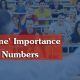# The Ratios; the fun of being one over the other###### How important are the AWA and IR Sections of the GMAT?
January 20, 2016###### The ‘Prime’ Importance of Prime Numbers
March 13, 2016

A commonly misunderstood concept in most competitive exams is the Ratio! Roughly, what we mean by ratio is something over something (x/y). So, we have a Numerator (the upper part of a fraction- in this case x) and a Denominator (the lower part of a fraction- in this case y). There is always a common multiple between the Numerator and the Denominator- irrespective of what the purpose is.

For eg: The ratio of the  ages of John (28 years) and Mary (18 years) = 28/12 = 14 x 2/9 x 2 = 14/9

In exams, we are given that ratio of John and Mary’s ages as 14/9 , and the most common error is that we assume John’s age to be 14 and Mary’s to be 9.

A typical example of data sufficiency question:

Is x > q ?

1. x/y = 5/3

2. x is positive.

In this question, the majority of the answers will be A (where, only statement 1 is sufficient to answer the question)

Let’s solve it.

Statement 1:

x/y = 5/3

As we have common multiples x = 5k and y = 3k

We do not know the exact value of k.

If k is positive, 5k > 3k , which means, x > y

If k is negative, 5k < 3k, which means, x < y

So, statement 1 is not giving us a unique answer, thus, A and D cannot be the answers.

Statement 2:

x is positive, it does not give us any information about q, so this statement is also not sufficient, thus, B cannot be the answer.

Combining 1 and 2:

Now, we know, x is positive. So 5k is positive, thus, k is positive.

Which gives a unique answer x > y.

Comparing the fractions:

If we need to check which fraction is bigger, the best method is to cross- multiply.

Eg: compare 5/7 and 2/3.

Multiply numerator of first fraction with the denominator of second fraction and multiply numerator of second fraction with denominator of second fraction.

So, 5 x 3 and 2 x 7,

We get, 15 and 14. As, 15 > 14, i.e. the first multiplication is bigger, so,

5/7 > 2/3.

Math is a scare for many who prepare for tests such as the GMAT and GRE. But is reality, the tests test math skills at a very basic level. What is intimidating about the math on these tests has more to do with understanding what the question asks rather than mustering up all one’s knowledge of math as a subject. Preparation then must be planned,focused and smart. One good way to get down to such training is to abandon the obsession with a score; don’t long to win your tryst with the GMAT; start instead by longing to learn- and remember a good learner is a sure winner.